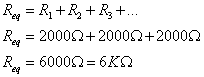# Regents Physics - Series Circuits

Developing an understanding of circuits is the first step in learning about the modern-day electronic devices that dominate what is becoming known as the "Information Age." A basic circuit type, the series circuit, is a circuit in which there is only a single current path. Kirchhoff's Laws provide us the tools in order to analyze any type of circuit.## Kirchhoff's Laws

Kirchhoff's Current Law (KCL), named after German physicist Gustav Kirchhoff, states that the sum of all current entering any point in a circuit has to equal the sum of all current leaving any point in a circuit. More simply, this is another way of looking at the law of conservation of charge.

Kirchhoff's Voltage Law (KVL) states that the sum of all the potential drops in any closed loop of a circuit has to equal zero. More simply, KVL is a method of applying the law of conservation of energy to a circuit.

Question: A 3.0-ohm resistor and a 6.0-ohm resistor are connected in series in an operating electric circuit. If the current through the 3.0-ohm resistor is 4.0 amperes, what is the potential difference across the 6.0-ohm resistor?Answer: First, let's draw a picture of the situation. If 4 amps of current is flowing through the 3-ohm resistor, then 4 amps of current must be flowing through the 6-ohm resistor according to Kirchhoff's Current Law. If we know the current and the resistance, we can calculate the voltage drop across the 6-ohm resistor using Ohm's Law:## Resistors in Series

Let's take a look at a sample circuit, consisting of three 2000-ohm (2K) resistors:There is only a single current path in the circuit, which travels through all three resistors. Instead of using three separate 2K resistors, we could replace the three resistors with one single resistor having an equivalent resistance. To find the equivalent resistance of any number of series resistors, we just add up their individual resistances:Note that because there is only a single current path, the same current must flow through each of the resistors.

## VIRP Tables

A simple and straightforward method for analyzing circuits involves creating a VIRP table for each circuit you encounter. Combining your knowledge of Ohm's Law, Kirchoff's Current Law, Kirchoff's Voltage Law, and equivalent resistance, you can use this table to solve for the details of any circuit.

A VIRP table describes the potential drop (V-voltage), current flow (I-current), resistance (R) and power dissipated (P-power) for each element in your circuit, as well as for the circuit as a whole. Let's use our circuit with the three 2000-ohm resistors as an example to demonstrate how a VIRP table is used. To create the VIRP table, we first list our circuit elements, and total, in the rows of the table, then make columns for V, I, R, and P:

VIRP Table
V I R P
R1
R2
R3
Total

Next, we fill in the information in the table that we know. For example, we know the total voltage in the circuit (12V) provided by the battery, and we know the values for resistance for each of the individual resistors:

VIRP Table
V I R P
R1     2000R2     2000R3     2000Total 12V

Once we have our initial information filled in, we can also calculate the total resistance, or equivalent resistance, of the entire circuit. In our case, this is 6000 ohms:

VIRP Table
V I R P
R1     2000R2     2000R3     2000Total 12V   6000If I look at the bottom (total) row of my table, I know both the voltage drop (V) and the resistance (R). Knowing these two items, I can calculate the total current flow in the circuit using Ohm's Law, and I can also calculate the total power dissipated in the circuit using my formulas for electrical power:I can now fill in more information in the VIRP table:

VIRP Table
V I R P
R1     2000R2     2000R3     2000Total 12V 0.002A 60000.024W

Because this is a series circuit, the total current has to be the same as the current through each individual element, so I can fill in the current through each of the individual resistors:

VIRP Table
V I R P
R1   0.002A 2000R2   0.002A 2000R3   0.002A 2000Total 12V 0.002A 60000.024W

Finally, for each element in the circuit I now know the current flow and the resistance. Using this knowledge, I can apply Ohm's Law to obtain the voltage drop (V=IR), and a formula for power (P=I2R) to complete the table.

VIRP Table
V I R P
R1 4V 0.002A 20000.008W
R2 4V 0.002A 20000.008W
R3 4V 0.002A 20000.008W
Total 12V 0.002A 60000.024W

So what does this table really tell us now that it's completely filled out? We know the potential drop across each resistor (4V), the current through each resistor (2 mA), and the power dissipated by each resistor (8 mW). In addition, we know the total potential drop for the entire circuit is 12V, and the entire circuit dissipated 24 mW of power. Note that for a series circuit, the sum of the individual voltage drops across each element equal the total potential difference in the circuit, the current is the same throughout the circuit, and the resistances and power dissipated values also add up to the total resistance and total power dissipated. These are summarized for you on your reference table as follows: